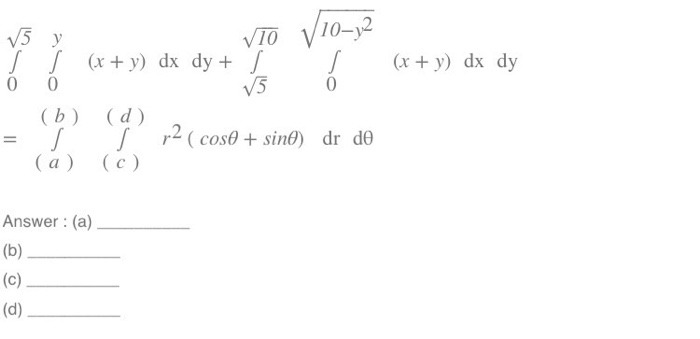# If x=a cos^3x and y=b sin^3x.find dy/dx?

If x=a cos^3x and y=b sin^3x.find dy/dx?

ReportAnswer 1

$y ' = - \frac{b}{a} \tan x$

#### Explanation:

We have that:

$\frac{x}{a \cos x} = {\cos}^{2} x$

and:

$\frac{y}{b \sin x} = {\sin}^{2} x$

Then:

$\frac{x}{a \cos x} + \frac{y}{b \sin x} = 1$

Differentiate implicitly:

$\frac{a \cos x + a x \sin x}{{a}^{2} {\cos}^{2} x} + \frac{b y ' \sin x - b y \cos x}{{b}^{2} {\sin}^{2} x} = 0$

$\frac{1}{a \cos x} + \frac{x \sin x}{a {\cos}^{2} x} + \frac{y '}{b \sin x} - \frac{y \cos x}{b {\sin}^{2} x} = 0$

$\frac{y '}{b \sin x} = \frac{y \cos x}{b {\sin}^{2} x} - \frac{x \sin x}{a {\cos}^{2} x} - \frac{1}{a \cos x}$

$y ' = \frac{y \cos x}{\sin x} - \frac{b x {\sin}^{2} x}{a {\cos}^{2} x} - \frac{b \sin x}{a \cos x}$

Substitute now $x$ and $y$ from the original equations:

$y ' = \frac{b {\sin}^{3} x \cos x}{\sin x} - \frac{a b {\cos}^{3} x {\sin}^{2} x}{a {\cos}^{2} x} - \frac{b \sin x}{a \cos x}$

and simplify:

$y ' = \cancel{b {\sin}^{2} x \cos x} - \cancel{b \cos x {\sin}^{2} x} - \frac{b \sin x}{a \cos x}$

Then:

$y ' = - \frac{b}{a} \tan x$

Similar Homework Help Questions
• ### Differential Equation dy/dx=sin(x)cos(y)

dy/dx=sin(x)cos(y)What is the solution to the equation at (0,(2n+1)π/2) where n is any integer?I know that you need to move common variables to the same side and then take the integral of both sides but I can't seem to be able to get y alone after that.Thanks.

• ### Find dy/dx for y=sin(3x+4y)

Find dy/dx for y=sin(3x+4y)

• ### Find dy/dx for the curve given by x= -sin(t) and y= cos(t)

Find dy/dx for the curve given by x= -sin(t) and y= cos(t)

• ### double integral: sin (x+y) dx dy

How do I integrate sin (x+y)? I know the answer is 0 because I used Maple. The "(x+y)" is really tripping me up. Anyone who can help is greatly appreciated.

• ### x dx dy + y) dx dy 0 (b (d a)(c) Answer: (a) x dx dy + y) dx dy 0 (b (d a)(c) Answer: (a)x dx dy + y) dx dy 0 (b (d a)(c) Answer: (a) x dx dy + y) dx dy 0 (b (d a)(c) Answer: (a)

• ### Find dy/dx of y=sin(cx)sin^c(x)#?

Free Homework App